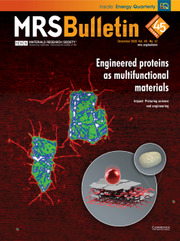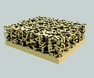Home
Hostname: page-component-55597f9d44-54vk6 Total loading time: 0.403 Render date: 2022-08-08T07:25:56.352Z Has data issue: true Feature Flags: { "shouldUseShareProductTool": true, "shouldUseHypothesis": true, "isUnsiloEnabled": true, "useRatesEcommerce": false, "useNewApi": true } hasContentIssue trueMRS Bulletin

# Architectural design and fabrication approaches for solid-state batteries

Published online by Cambridge University Press:  10 October 2018

## AbstractSolid-state batteries are promising candidates for energy storage due to their potential advantages in safety, working temperature range, and energy density compared to traditional liquid-electrolyte-based batteries. Rational battery architecture design and a scalable fabrication approach are critical to realize solid-state batteries. In this article, we present the architecture, fabrication procedure, and related challenges of sulfide and oxide electrolyte-based solid-state batteries. Approaches toward intimate solid−solid contact, thin solid-electrolyte fabrication, and scale-up production are discussed. Finally, we discuss the future research directions of solid-state batteries.

## Keywords

Type
Frontiers of Solid-State Batteries
Information
MRS Bulletin , October 2018 , pp. 775 - 781

## Access options

Get access to the full version of this content by using one of the access options below. (Log in options will check for institutional or personal access. Content may require purchase if you do not have access.)

## References

Janek, J., Zeier, W.G., Nat. Energy 1, 16141 (2016).CrossRefGoogle Scholar
Mikhaylik, Y.V., Akridge, J.R., J. Electrochem. Soc. 151, A1969 (2004).CrossRefGoogle Scholar
Luntz, A.C., Voss, J., Reuter, K., J. Phys. Chem. Lett. 6, 4599 (2015).CrossRefGoogle Scholar
Kato, Y., Hori, S., Saito, T., Suzuki, K., Hirayama, M., Mitsui, A., Yonemura, M., Iba, H., Kanno, R., Nat. Energy 1, 16030 (2016).CrossRefGoogle Scholar
Kato, Y., Shiotani, S., Morita, K., Suzuki, K., Hirayama, M., Kanno, R., J. Phys. Chem. Lett. 9, 607 (2018).CrossRefGoogle Scholar
Schnell, J., Günther, T., Knoche, T., Vieider, C., Köhler, L., Just, A., Keller, M., Passerini, S., Reinhart, G., J. Power Sources 382, 160 (2018).CrossRefGoogle Scholar
Bachman, J.C., Muy, S., Grimaud, A., Chang, H.-H., Pour, N., Lux, S.F., Paschos, O., Maglia, F., Lupart, S., Lamp, P., Giordano, L., Shao-Horn, Y., Chem. Rev. 116, 140 (2016).CrossRefGoogle Scholar
Thangadurai, V., Narayanan, S., Pinzaru, D., Chem. Soc. Rev. 43, 4714 (2014).CrossRefGoogle Scholar
Han, F., Zhu, Y., He, X., Mo, Y., Wang, C., Adv. Energy Mater. 6, 1501590 (2016).CrossRefGoogle Scholar
Koerver, R., Aygün, I., Leichtweiß, T., Dietrich, C., Zhang, W., Binder, J.O., Hartmann, P., Zeier, W.G., Janek, J., Chem. Mater. 29, 5574 (2017).CrossRefGoogle Scholar
Ren, Y., Shen, Y., Lin, Y., Nan, C.-W., Electrochem. Commun. 57, 27 (2015).CrossRefGoogle Scholar
Cheng, E.J., Sharafi, A., Sakamoto, J., Electrochim. Acta 223, 85 (2017).CrossRefGoogle Scholar
Han, F., Yue, J., Zhu, X., Wang, C., Adv. Energy Mater. 8, 1703644 (2018).CrossRefGoogle Scholar
Kerman, K., Luntz, A., Viswanathan, V., Chiang, Y.-M., Chen, Z., J. Electrochem. Soc. 164, A1731 (2017).CrossRefGoogle Scholar
Knoche, T., Zinth, V., Schulz, M., Schnell, J., Gilles, R., Reinhart, G., J. Power Sources 331, 267 (2016).CrossRefGoogle Scholar
Kamaya, N., Homma, K., Yamakawa, Y., Hirayama, M., Kanno, R., Yonemura, M., Kamiyama, T., Kato, Y., Hama, S., Kawamoto, K., Mitsui, A., Nat. Mater. 10, 682 (2011).CrossRefGoogle Scholar
Han, F., Yue, J., Chen, C., Zhao, N., Fan, X., Ma, Z., Gao, T., Wang, F., Guo, X., Wang, C., Joule 2, 497 (2018).CrossRefGoogle Scholar
Zhang, W., Leichtweiß, T., Culver, S.P., Koerver, R., Das, D., Weber, D.A., Zeier, W.G., Janek, J., ACS Appl. Mater. Interfaces 9, 35888 (2017).CrossRefGoogle ScholarPubMed
Monroe, C., Newman, J., J. Electrochem. Soc. 152, A396 (2005).CrossRefGoogle Scholar
Neudecker, B.J., Dudney, N.J., Bates, J.B., J. Electrochem. Soc. 147, 517 (2000).CrossRefGoogle Scholar
Porz, L., Swamy, T., Sheldon, B.W., Rettenwander, D., Frömling, T., Thaman, H.L., Berendts, S., Uecker, R., Carter, W.C., Chiang, Y.-M., Adv. Energy Mater. 7, 1701003 (2017).CrossRefGoogle Scholar
Sakuda, A., Hayashi, A., Tatsumisago, M., Sci. Rep. 3, 2261 (2013).CrossRefGoogle Scholar
Yu, S., Schmidt, R.D., Garcia-Mendez, R., Herbert, E., Dudney, N.J., Wolfenstine, J.B., Sakamoto, J., Siegel, D.J., Chem. Mater. 28, 197 (2016).CrossRefGoogle Scholar
Nagao, M., Hayashi, A., Tatsumisago, M., Electrochim. Acta 56, 6055 (2011).CrossRefGoogle Scholar
Nagao, M., Hayashi, A., Tatsumisago, M., J. Mater. Chem. 22, 10015 (2012).CrossRefGoogle Scholar
Choi, H.U., Jin, J.S., Park, J.-Y., Lim, H.-T., J. Alloys Compd. 723, 787 (2017).CrossRefGoogle Scholar
Liu, Z., Fu, W., Payzant, E.A., Yu, X., Wu, Z., Dudney, N.J., Kiggans, J., Hong, K., Rondinone, A.J., Liang, C., J. Am. Chem. Soc. 135, 975 (2013).CrossRefGoogle Scholar
Lin, Z., Liu, Z., Dudney, N.J., Liang, C., ACS Nano 7, 2829 (2013).CrossRefGoogle Scholar
Teragawa, S., Aso, K., Tadanaga, K., Hayashi, A., Tatsumisago, M., J. Mater. Chem. A 2, 5095 (2014).CrossRefGoogle Scholar
Choi, Y.E., Park, K.H., Kim, D.H., Oh, D.Y., Kwak, H.R., Lee, Y.-G., Jung, Y.S., ChemSusChem 10, 2605 (2017).CrossRefGoogle Scholar
Kim, D.H., Oh, D.Y., Park, K.H., Choi, Y.E., Nam, Y.J., Lee, H.A., Lee, S.-M., Jung, Y.S., Nano Lett . 17, 3013 (2017).CrossRefGoogle Scholar
Han, F., Yue, J., Fan, X., Gao, T., Luo, C., Ma, Z., Suo, L., Wang, C., Nano Lett . 16, 4521 (2016).CrossRefGoogle Scholar
Huo, H., Zhao, N., Sun, J., Du, F., Li, Y., Guo, X., J. Power Sources 372, 1 (2017).CrossRefGoogle Scholar
Ohta, S., Seki, J., Yagi, Y., Kihira, Y., Tani, T., Asaoka, T., J. Power Sources 265, 40 (2014).CrossRefGoogle Scholar
Park, K., Yu, B.-C., Jung, J.-W., Li, Y., Zhou, W., Gao, H., Son, S., Goodenough, J.B., Chem. Mater. 28, 8051 (2016).CrossRefGoogle Scholar
Aboulaich, A., Bouchet, R., Delaizir, G., Seznec, V., Tortet, L., Morcrette, M., Rozier, P., Tarascon, J.-M., Viallet, V., Dollé, M., Adv. Energy Mater. 1, 179 (2011).CrossRefGoogle Scholar
Kato, T., Hamanaka, T., Yamamoto, K., Hirayama, T., Sagane, F., Motoyama, M., Iriyama, Y., J. Power Sources 260, 292 (2014).CrossRefGoogle Scholar
Zhou, W., Wang, S., Li, Y., Xin, S., Manthiram, A., Goodenough, J.B., J. Am. Chem. Soc. 138, 9385 (2016).CrossRefGoogle Scholar
Ren, Y., Liu, T., Shen, Y., Lin, Y., Nan, C.-W., Ionics 23, 2521 (2017).CrossRefGoogle Scholar
van den Broek, J., Afyon, S., Rupp, J.L.M., Adv. Energy Mater. 6, 1600736 (2016).CrossRefGoogle Scholar
Gong, Y., Fu, K., Xu, S., Dai, J., Hamann, T.R., Zhang, L., Hitz, G.T., Fu, Z., Ma, Z., McOwen, D.W., Han, X., Hu, L., Wachsman, E.D., Mater. Today 21, 594 (2018).CrossRefGoogle Scholar
Fu, K., Gong, Y., Hitz, G.T., McOwen, D.W., Li, Y., Xu, S., Wen, Y., Zhang, L., Wang, C., Pastel, G., Dai, J., Liu, B., Xie, H., Yao, Y., Wachsman, E.D., Hu, L., Energy Environ. Sci. 10, 1568 (2017).CrossRefGoogle Scholar
Han, X., Gong, Y., Fu, K., He, X., Hitz, G.T., Dai, J., Pearse, A., Liu, B., Wang, H., Rubloff, G., Mo, Y., Thangadurai, V., Wachsman, E.D., Hu, L., Nat. Mater. 16, 572 (2016).CrossRefGoogle Scholar
Yang, C., Zhang, L., Liu, B., Xu, S., Hamann, T., McOwen, D., Dai, J., Luo, W., Gong, Y., Wachsman, E.D., Hu, L., Proc. Natl. Acad. Sci. U.S.A. 115, 3770 (2018).CrossRefGoogle Scholar
Wu, B., Wang, S., Evans IV, W.J., Deng, D.Z., Yang, J., Xiao, J., J. Mater. Chem. A 4, 15266 (2016).CrossRefGoogle Scholar
McCloskey, B.D., J. Phys. Chem. Lett. 6, 4581 (2015).CrossRefGoogle Scholar
Kim, S., Hirayama, M., Taminato, S., Kanno, R., Dalton Trans . 42, 13112 (2013).CrossRefGoogle Scholar
Chen, R.-J., Huang, M., Huang, W.-Z., Shen, Y., Lin, Y.-H., Nan, C.-W., J. Mater. Chem. A 2, 13277 (2014).CrossRefGoogle Scholar
Ahn, C.-W., Choi, J.-J., Ryu, J., Hahn, B.-D., Kim, J.-W., Yoon, W.-H., Choi, J.-H., Park, D.-S., J. Electrochem. Soc. 162, A60 (2015).CrossRefGoogle Scholar
Lobe, S., Dellen, C., Finsterbusch, M., Gehrke, H.G., Sebold, D., Tsai, C.L., Uhlenbruck, S., Guillon, O., J. Power Sources 307, 684 (2016).CrossRefGoogle Scholar
Kazyak, E., Chen, K.-H., Wood, K.N., Davis, A.L., Thompson, T., Bielinski, A.R., Sanchez, A.J., Wang, X., Wang, C., Sakamoto, J., Dasgupta, N.P., Chem. Mater. 29, 3785 (2017).CrossRefGoogle Scholar
Yi, E., Wang, W., Kieffer, J., Laine, R.M., J. Mater. Chem. A 4, 12947 (2016).CrossRefGoogle Scholar
Inada, T., Kobayashi, T., Sonoyama, N., Yamada, A., Kondo, S., Nagao, M., Kanno, R., J. Power Sources 194, 1085 (2009).CrossRefGoogle Scholar
Nam, Y.J., Cho, S.-J., Oh, D.Y., Lim, J.-M., Kim, S.Y., Song, J.H., Lee, Y.-G., Lee, S.-Y., Jung, Y.S., Nano Lett . 15, 3317 (2015).CrossRefGoogle Scholar
Sakuda, A., Kuratani, K., Yamamoto, M., Takahashi, M., Takeuchi, T., Kobayashi, H., J. Electrochem. Soc. 164, A2474 (2017).CrossRefGoogle Scholar
Yamamoto, M., Terauchi, Y., Sakuda, A., Takahashi, M., Sci. Rep. 8, 1212 (2018).CrossRefGoogle Scholar
Lee, K., Kim, S., Park, J., Park, S.H., Coskun, A., Jung, D.S., Cho, W., Choi, J.W., J. Electrochem. Soc. 164, A2075 (2017).CrossRefGoogle Scholar
Oh, D.Y., Kim, D.H., Jung, S.H., Han, J.-G., Choi, N.-S., Jung, Y.S., J. Mater. Chem. A 5, 20771 (2017).CrossRefGoogle Scholar
Ong, S.P., Mo, Y., Richards, W.D., Miara, L., Lee, H.S., Ceder, G., Energy Environ. Sci. 6, 148 (2013).CrossRefGoogle Scholar
Olivetti, E.A., Ceder, G., Gaustad, G.G., Fu, X., Joule 1, 229 (2017).CrossRefGoogle Scholar
Liang, Y., Jing, Y., Gheytani, S., Lee, K.-Y., Liu, P., Facchetti, A., Yao, Y., Nat. Mater. 16, 841 (2017).CrossRefGoogle Scholar
Chi, X., Liang, Y., Hao, F., Zhang, Y., Whiteley, J., Dong, H., Hu, P., Lee, S., Yao, Y., Angew. Chem. Int. Ed. Engl. 57, 2630 (2018).CrossRefGoogle Scholar
Bucci, G., Swamy, T., Chiang, Y.-M., Carter, W.C., J. Mater. Chem. A 5, 19422 (2017).CrossRefGoogle Scholar
Chen, R.-J., Zhang, Y.-B., Liu, T., Xu, B.-Q., Lin, Y.-H., Nan, C.-W., Shen, Y., ACS Appl. Mater. Interfaces 9, 9654 (2017).CrossRefGoogle Scholar
Wang, Y., Lai, W., J. Power Sources 275, 612 (2015).CrossRefGoogle Scholar
Cheng, L., Crumlin, E.J., Chen, W., Qiao, R., Hou, H., Lux, S.F., Zorba, V., Russo, R., Kostecki, R., Liu, Z., Persson, K., Yang, W., Cabana, J., Richardson, T., Chen, G., Doeff, M., Phys. Chem. Chem. Phys. 16, 18294 (2014).CrossRefGoogle Scholar
Sharafi, A., Kazyak, E., Davis, A.L., Yu, S., Thompson, T., Siegel, D.J., Dasgupta, N.P., Sakamoto, J., Chem. Mater. 29, 7961 (2017).CrossRefGoogle Scholar
Li, Y., Chen, X., Dolocan, A., Cui, Z., Xin, S., Xue, L., Xu, H., Park, K., Goodenough, J.B., J. Am. Chem. Soc. 140, 6448 (2018).CrossRefGoogle Scholar
Li, Y., Wang, Z., Li, C., Cao, Y., Guo, X., J. Power Sources 248, 642 (2014).CrossRefGoogle Scholar
Ito, S., Fujiki, S., Yamada, T., Aihara, Y., Park, Y., Kim, T.Y., Baek, S.-W., Lee, J.-M., Doo, S., Machida, N., J. Power Sources 248, 943 (2014).CrossRefGoogle Scholar
Inada, T., Takada, K., Kajiyama, A., Sasaki, H., Kondo, S., Watanabe, M., Murayama, M., Kanno, R., J. Power Sources 119−121, 948 (2003).CrossRefGoogle Scholar
Menzler, N.H., Tietz, F., Uhlenbruck, S., Buchkremer, H.P., Stöver, D., J. Mater. Sci. 45, 3109 (2010).CrossRefGoogle Scholar

# Save article to Kindle

Note you can select to save to either the @free.kindle.com or @kindle.com variations. ‘@free.kindle.com’ emails are free but can only be saved to your device when it is connected to wi-fi. ‘@kindle.com’ emails can be delivered even when you are not connected to wi-fi, but note that service fees apply.

Find out more about the Kindle Personal Document Service.

Architectural design and fabrication approaches for solid-state batteries
Available formats
×

# Save article to Dropbox

To save this article to your Dropbox account, please select one or more formats and confirm that you agree to abide by our usage policies. If this is the first time you used this feature, you will be asked to authorise Cambridge Core to connect with your Dropbox account. Find out more about saving content to Dropbox.

Architectural design and fabrication approaches for solid-state batteries
Available formats
×

# Save article to Google Drive

To save this article to your Google Drive account, please select one or more formats and confirm that you agree to abide by our usage policies. If this is the first time you used this feature, you will be asked to authorise Cambridge Core to connect with your Google Drive account. Find out more about saving content to Google Drive.

Architectural design and fabrication approaches for solid-state batteries
Available formats
×
×